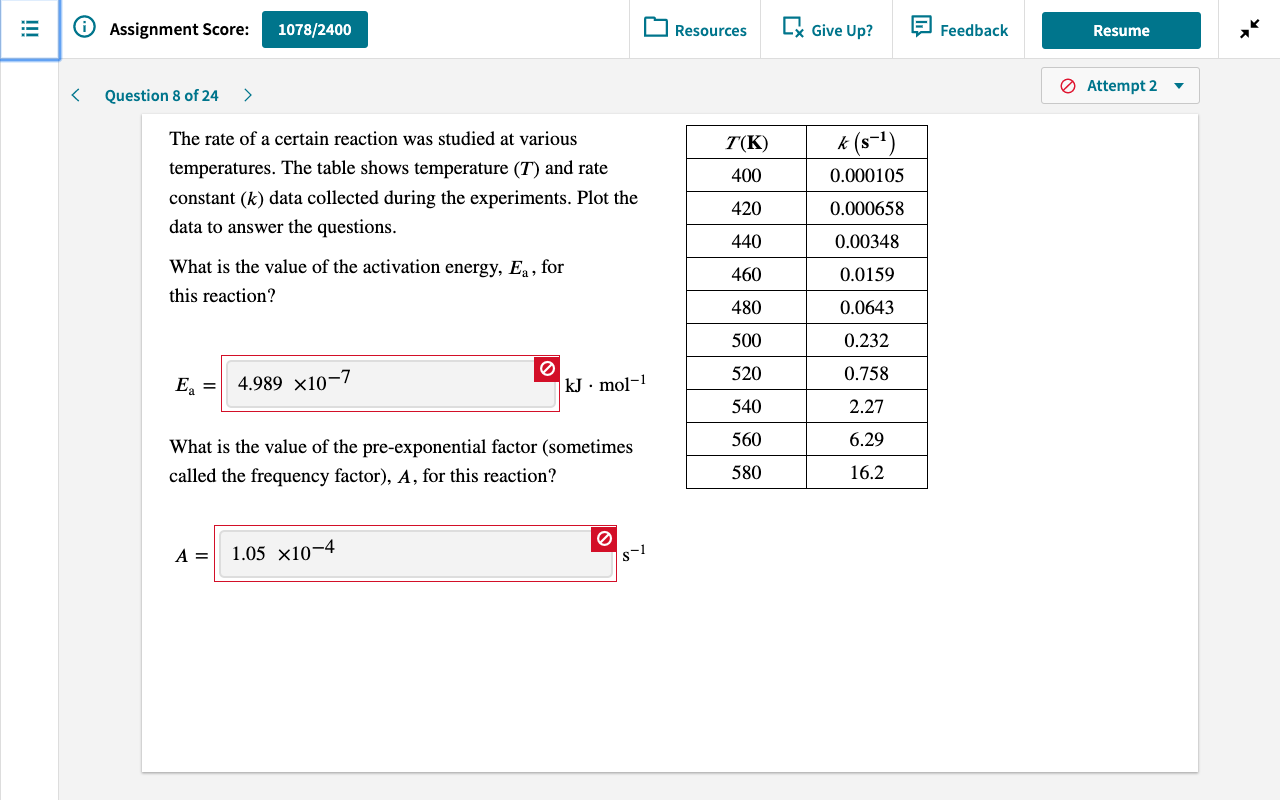# The rate of a certain reaction was studied at various temperatures. The table shows temperature (T) and rate constant (k) data collected during the experiments. Plot the data to answer the questions. What is the Activation Energy, Ea, for this reaction? What is the value of the pre-exponential factor (sometimes called frequency factor), A, for this reaction?